# Create a Sleek Vertical Business Card in InDesign

So, today,we are going to create an appealing vertical business card in InDesign. We will use some useful techniques, tools, and tricks to get this done. Let’s get stated.### Step 1

Open InDesign and click on “File” > “New” > “Document,” with the number of pages set at 2. Uncheck the Facing pages. You can use the size that your printer prefers; I’m going to set the width to 2in and the height to 3.5in with margins of 0.5in and a bleed 0.125in.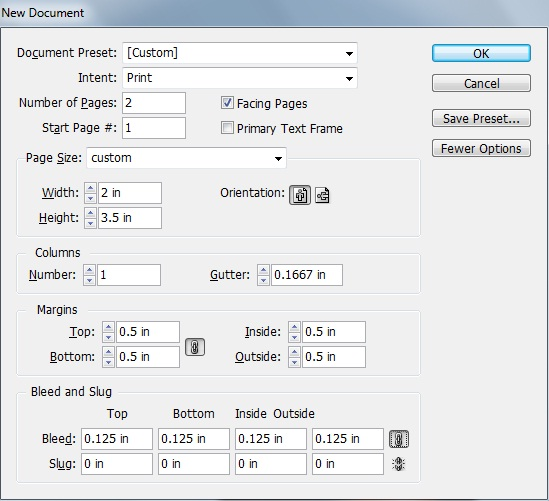### Step 2

Now, select the rectangle tool and create a rectangle with a dark color swatch (C=0 M=0 Y=0 K=92).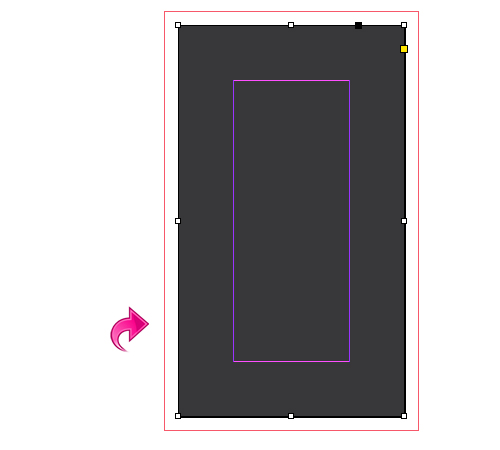### Step 3

Select the line tool and create a vertical line with the same color used in the above rectangle. Then, add a 4pt “white diamond” stroke with color swatch (C=0 M=0 Y=0 K=93).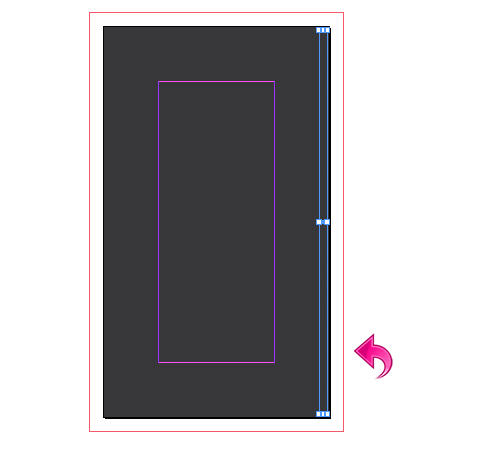### Step 4

Repeat the same process and fill the background with these lines.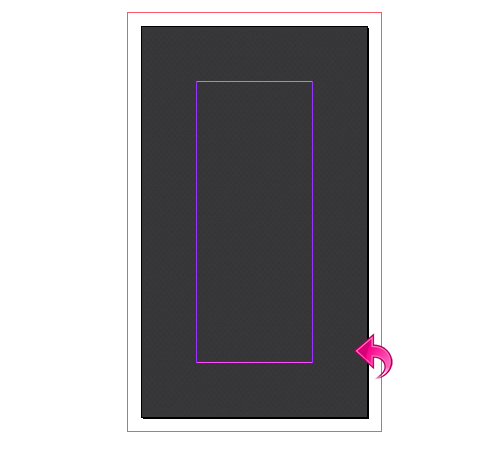### Step 5

Before we start with the design elements, let’s change the screen mode to “Preview” so that we can properly see the details. Now, create a new layer (using the layer panel) then select the pen tool and create a path like the one below. Fill it with color swatch (C=9 M=75 Y=100 K=7).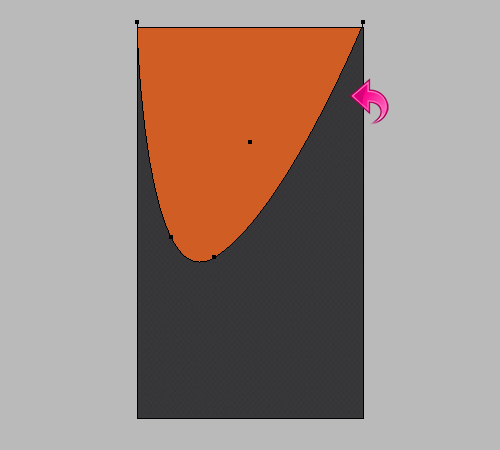### Step 6

Select the above layer and click on effects to add some drop shadow. Use the following settings.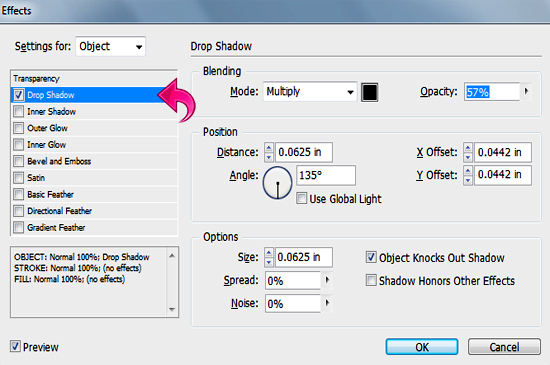### Step 7

Select the pen tool again, create another path, and fill it with paper color swatch (C=0 M=0 Y=0 K=0). Then, click on “Effects” and add a drop shadow using the same values used before.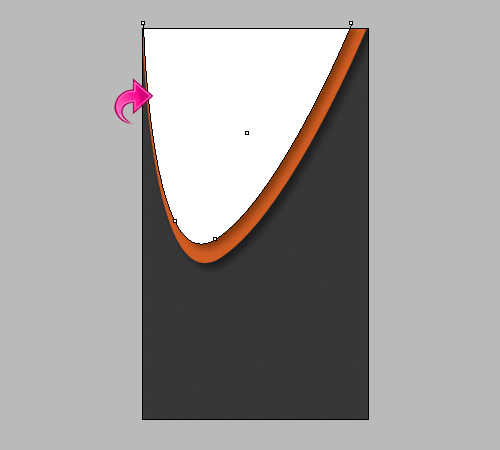### Step 8

Repeat the same process and create some more paths with similar colors and drop shadows.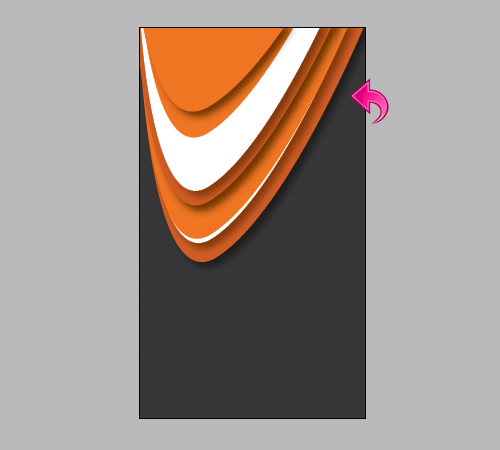### Step 9

Now, let’s start with bottom. Select the pen tool, create a path, and fill it with color swatch (C=9 M=75 Y=100 K=7).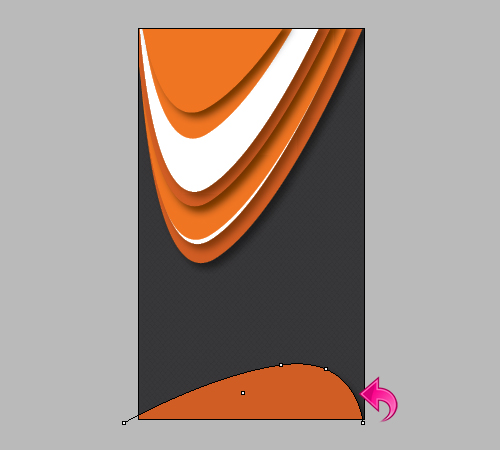### Step 10

Create two more paths with colors (C=9 M=75 Y=100 K=7) and (C=1 M=67 Y=100 K=0). Then, click on “Effects” to add some drop shadow. Use the values shown below.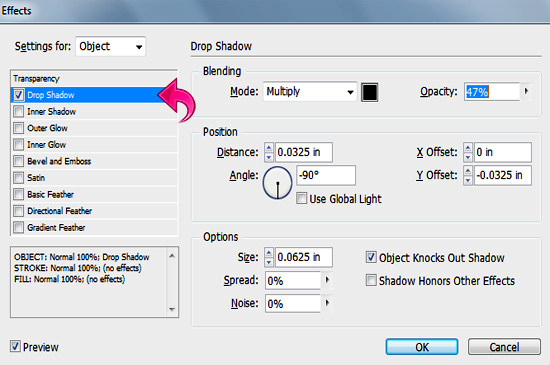### Step 11

Now, let’s add the title text. Select the type tool and add text using paper swatch (C=0 M=0 Y=0 K=0).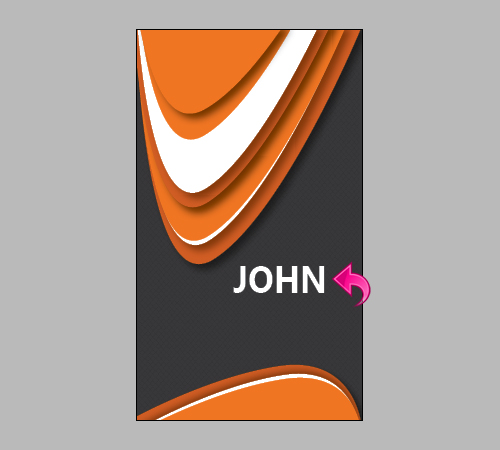### Step 12

Select the above layer and click on “Effects” to add a drop shadow and inner shadow using the following settings.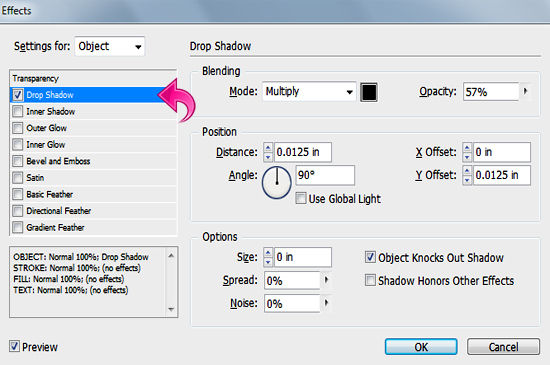### Step 13

Repeat the same process and add some more text. We’re done with the front side!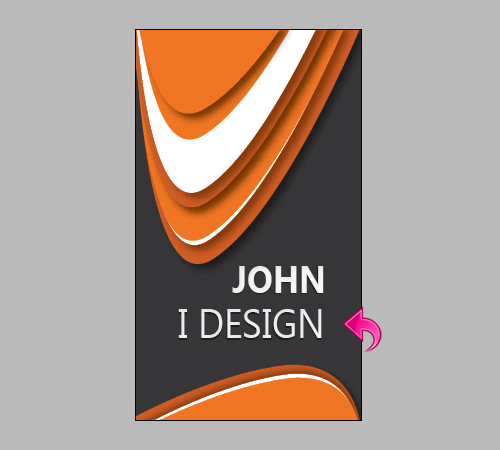### Step 14

Now, let’s start with the other side. Create a new layer and select the rectangle tool to create a rectangle using the same printer-friendly dimensions that you chose before.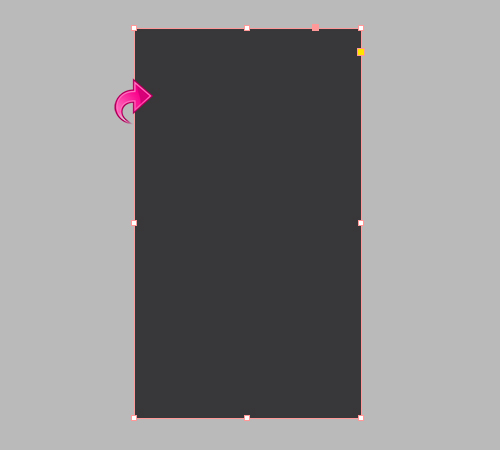### Step 15

Select the line tool to add lines with a 4pt “White diamond” stroke, the same way that we did above.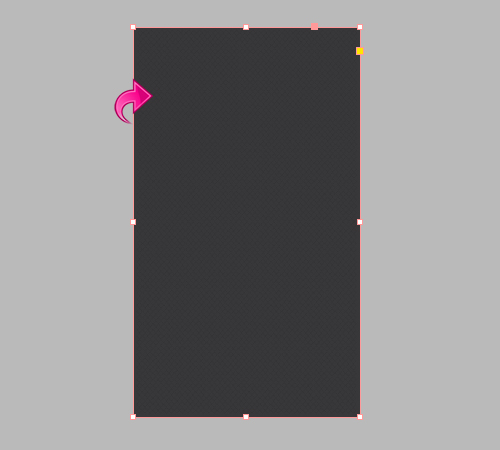### Step 16

Select the pen tool to create a path and fill it with color swatch (C=1 M=67 Y=100 K=0).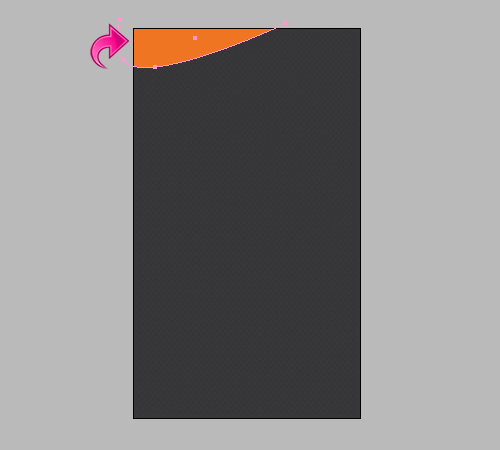### Step 17

Repeat the same process and create rest of the paths and add some drop shadow to each.### Step 18

Select the type tool, add text with a pure white paper swatch, and add inner shadow and drop shadow. Use the same settings that we used for the text on the other side of the card.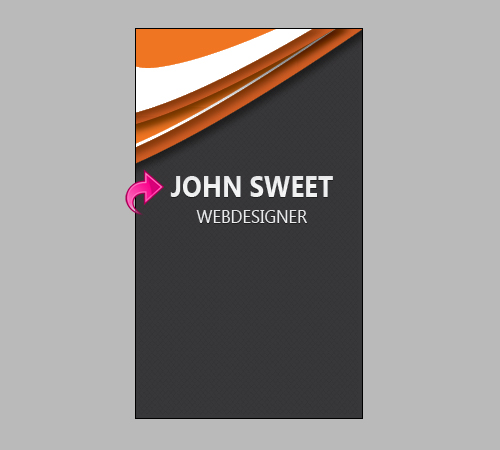### Step 19### Step 20

Now, select the line tool and create a divider between the texts. Add a 3pt “White diamond” stroke to line with a gray color swatch (C=0 M=0 Y=0 K=83).### Step 21

Select the rectangle tool and create a small rectangle with color (C=0 M=0 Y=0 K=94).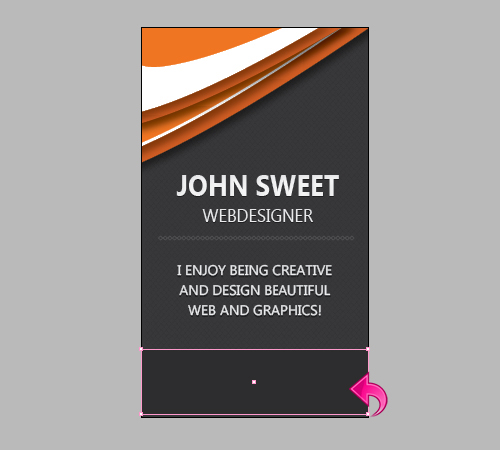### Step 22

Now, select the type tool and add the contact details into the design.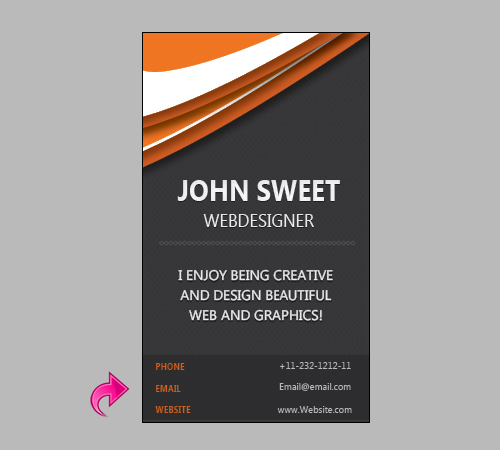### Step 23

Select the line tool and create dividers with a 1pt stroke using a dark color swatch (C=0 M=0 Y=0 K=93).And, we are done with our vertical business card. Looking sharp! I hope you enjoyed the process.